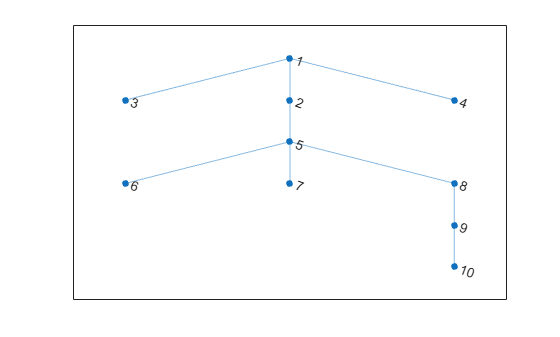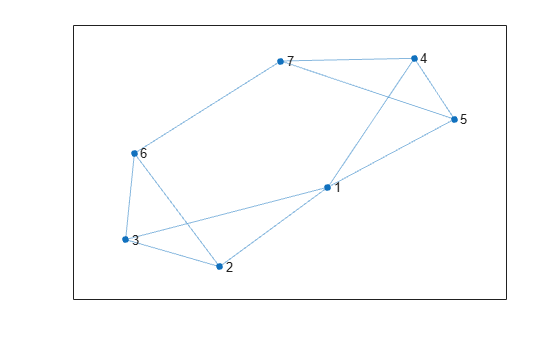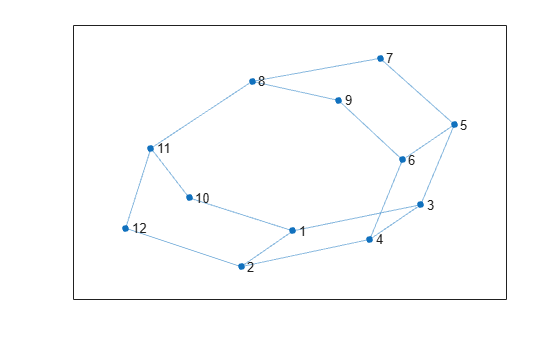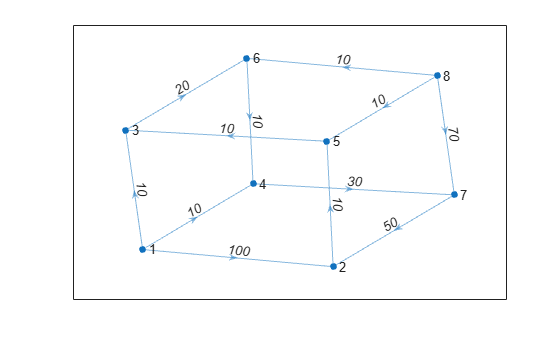# distances

## 语法

``d = distances(G)``
``d = distances(G,s)``
``d = distances(G,s,t)``
``d = distances(___,'Method',algorithm)``

## 说明

``d = distances(G)` 返回矩阵 `d`，其中 `d(i,j)` 是节点 `i` 和节点 `j` 之间的最短路径的长度。如果图进行了加权（即 `G.Edges` 包含变量 `Weight`），则这些权重用作沿图中各边的距离。否则，所有边距离都视为 `1`。`

``d = distances(G,s)` 将源节点限制为由 `s` 定义的节点，这样 `d(i,j)` 就是从节点 `s(i)` 到节点 `j` 的距离。`

``d = distances(G,s,t)` 还将目标节点限制为由 `t` 定义的节点，这样 `d(i,j)` 就是从节点 `s(i)` 到节点 `t(j)` 的距离。`

``d = distances(___,'Method',algorithm)` 可以使用上述语法中的任何输入参数指定在计算最短路径时使用的算法。例如，如果 `G` 是加权图，则 `distances(G,'Method','unweighted')` 将忽略 `G` 中的边权重，而将所有边权重视为 `1`。`

## 示例

```s = [1 1 1 2 5 5 5 8 9]; t = [2 3 4 5 6 7 8 9 10]; G = graph(s,t); plot(G)````d = distances(G)`
```d = 10×10 0 1 1 1 2 3 3 3 4 5 1 0 2 2 1 2 2 2 3 4 1 2 0 2 3 4 4 4 5 6 1 2 2 0 3 4 4 4 5 6 2 1 3 3 0 1 1 1 2 3 3 2 4 4 1 0 2 2 3 4 3 2 4 4 1 2 0 2 3 4 3 2 4 4 1 2 2 0 1 2 4 3 5 5 2 3 3 1 0 1 5 4 6 6 3 4 4 2 1 0 ```

`d` 是对称的，因为 `G` 为无向图。通常，`d(i,j)` 是节点 `i` 和节点 `j` 之间的最短路径长度，对于无向图，它等于 `d(j,i)`

`d(1,10)`
```ans = 5 ```

```s = [1 1 1 1 2 2 3 4 4 5 6]; t = [2 3 4 5 3 6 6 5 7 7 7]; G = graph(s,t); plot(G)````d = distances(G,[1 2 3])`
```d = 3×7 0 1 1 1 1 2 2 1 0 1 2 2 1 2 1 1 0 2 2 1 2 ```

`d(1,7)`
```ans = 2 ```

```s = [1 1 1 2 2 3 3 4 5 5 6 7 8 8 10 11]; t = [2 3 10 4 12 5 4 6 6 7 9 8 9 11 11 12]; G = graph(s,t); plot(G)``````sources = [5 7]; targets = [2 3]; d = distances(G,sources,targets)```
```d = 2×2 3 1 4 2 ```

`d(2,2)`
```ans = 2 ```

```s = [1 1 1 2 5 3 6 4 7 8 8 8]; t = [2 3 4 5 3 6 4 7 2 6 7 5]; weights = [100 10 10 10 10 20 10 30 50 10 70 10]; G = digraph(s,t,weights); plot(G,'EdgeLabel',G.Edges.Weight)````d = distances(G)`
```d = 8×8 0 90 10 10 100 30 40 Inf Inf 0 20 50 10 40 80 Inf Inf 110 0 30 120 20 60 Inf Inf 80 100 0 90 120 30 Inf Inf 120 10 40 0 30 70 Inf Inf 90 110 10 100 0 40 Inf Inf 50 70 100 60 90 0 Inf Inf 100 20 20 10 10 50 0 ```

`d1 = distances(G,'Method','unweighted')`
```d1 = 8×8 0 1 1 1 2 2 2 Inf Inf 0 2 4 1 3 5 Inf Inf 4 0 2 5 1 3 Inf Inf 2 4 0 3 5 1 Inf Inf 5 1 3 0 2 4 Inf Inf 3 5 1 4 0 2 Inf Inf 1 3 5 2 4 0 Inf Inf 2 2 2 1 1 1 0 ```

## 输入参数

`s``t` 不得指定名为 `'all'``'Method'` 的节点，因为这些节点名称与选项名称冲突。对于这些情况，请改用 `findnode` 传入节点索引。

`s``t` 不得指定名为 `'all'``'Method'` 的节点，因为这些节点名称与选项名称冲突。对于这些情况，请改用 `findnode` 传入节点索引。

`'auto'`（默认值）

`'auto'` 选项会自动选择算法：

• `'unweighted'` 用于没有边权重的 `graph``digraph` 输入。

• `'positive'` 用于具有边权重的所有 `graph` 输入，并要求权重为非负数。此选项还用于具有非负边权重的 `digraph` 输入。

• `'mixed'` 用于其边权重包含某些负值的 `digraph` 输入。图不能包含负循环。

`'unweighted'`

`'positive'`

Dijkstra 算法，要求所有边权重均为非负数。

`'mixed'`（仅适用于 `digraph`

## 提示

• `shortestpath``shortestpathtree``distances` 函数不支持具有负边权重的无向图，或更通俗地说，不支持包含负循环的任何图，原因如下：

• 负循环是从节点出发回到自身的路径，路径上的边权重之和为负值。如果两个节点之间的路径上具有负循环，则这两个节点之间不存在最短路径，因为始终可以通过遍历负循环找到更短路径。

• 无向图中的单个负边权重会创建一个负循环。